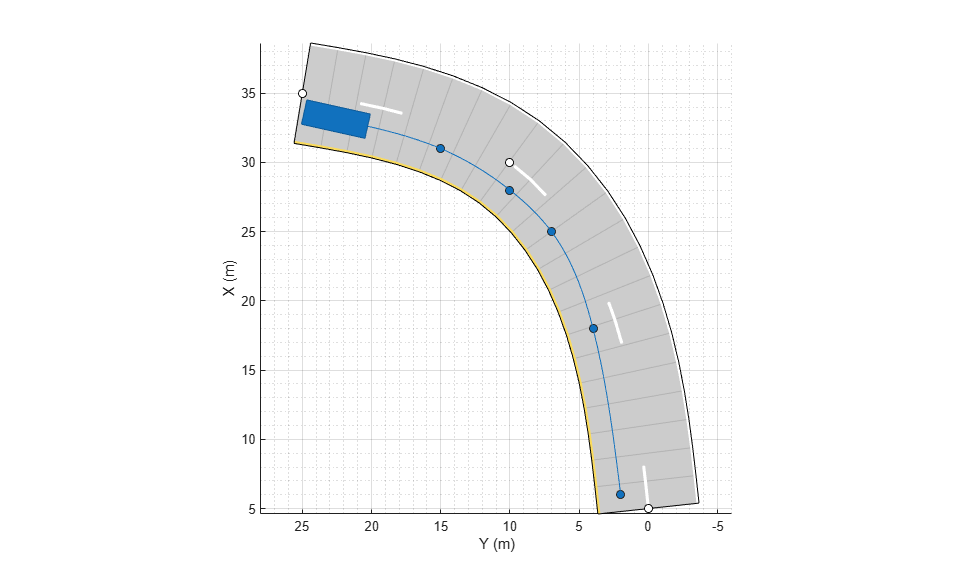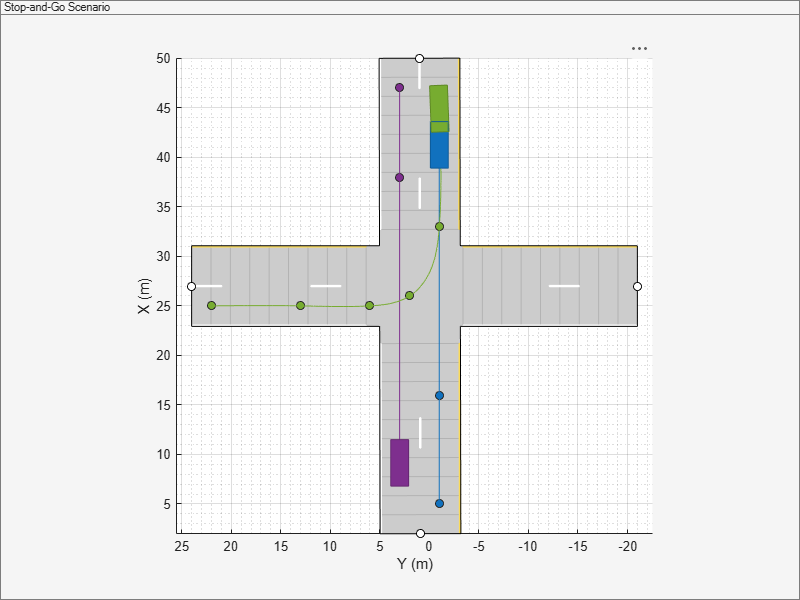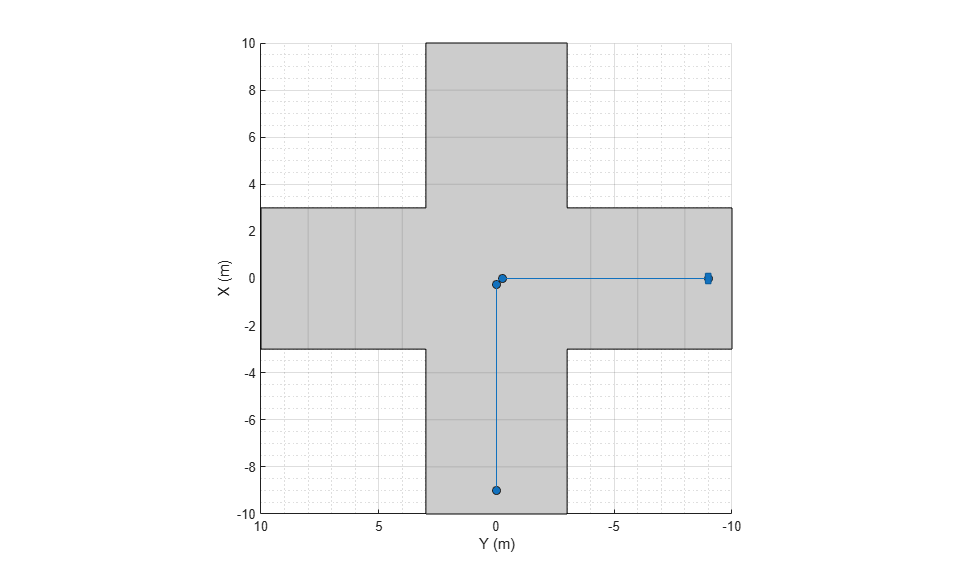# trajectory

Create actor or vehicle trajectory in driving scenario

## Syntax

``trajectory(ac,waypoints)``
``trajectory(ac,waypoints,speed)``
``trajectory(ac,waypoints,speed,waittime)``
``trajectory(___,'Yaw',yaw)``

## Description

````trajectory(ac,waypoints)` creates a trajectory for an actor or vehicle, `ac`, from a set of waypoints. NoteThe trajectory function generates trajectories that have discontinuities in acceleration between waypoints, resulting in high amounts of jerk. To generate a smooth, jerk-limited trajectory, use the `smoothTrajectory` function instead. ```

example

````trajectory(ac,waypoints,speed)` also specifies the speed with which the actor or vehicle travels along the trajectory, in either forward or reverse motion.```

example

````trajectory(ac,waypoints,speed,waittime)` specifies the wait time for an actor or vehicle in addition to the input arguments in the previous syntax. Use this syntax to generate stop-and-go driving scenarios by pausing an actor or vehicle actors at specific waypoints.```

example

````trajectory(___,'Yaw',yaw)` specifies the yaw orientation angle of the actor or vehicle at each waypoint, in addition to any of the input argument combinations from preceding syntaxes.```

## Examples

collapse all

Create a driving scenario and add a curved two-lane road to it.

```scenario = drivingScenario('SampleTime',0.05); roadcenters = [5 0; 30 10; 35 25]; lspec = lanespec(2); road(scenario,roadcenters,'Lanes',lspec); ```

Add a vehicle to the scenario. Set a trajectory in which the vehicle drives around the curve at varying speeds.

```v = vehicle(scenario,'ClassID',1); waypoints = [6 2; 18 4; 25 7; 28 10; 31 15; 33 22]; speeds = [30 10 5 5 10 30]; trajectory(v,waypoints,speeds) ```

Plot the scenario and run the simulation. Observe how the vehicle slows down as it drives along the curve.

```plot(scenario,'Waypoints','on','RoadCenters','on') while advance(scenario) pause(0.1) end ```Create a driving scenario consisting of two, two-lane roads that intersect at a right angle.

```scenario = drivingScenario('StopTime',2.75); roadCenters = [50 1 0; 2 0.9 0]; laneSpecification = lanespec(2,'Width',4); road(scenario,roadCenters,'Lanes',laneSpecification); roadCenters = [27 24 0; 27 -21 0]; road(scenario,roadCenters,'Lanes',laneSpecification);```

Add an ego vehicle to the scenario. Specify the waypoints and the speed values for the vehicle at each waypoint. Set a wait time for the vehicle at the second waypoint. Generate a trajectory in which the ego vehicle travels through the specified waypoints at the specified speed.

```egoVehicle = vehicle(scenario,'ClassID',1,'Position',[5 -1 0]); waypoints = [5 -1 0; 16 -1 0; 40 -1 0]; speed = [30; 0; 30]; waittime = [0; 0.3; 0]; trajectory(egoVehicle,waypoints,speed,waittime);```

Add a car to the scenario. Specify the waypoints and the speed values for the car at each waypoint. Set a wait time for the car at the second waypoint. Generate a trajectory in which the car travels through the specified waypoints at the specified speed.

```car = vehicle(scenario,'ClassID',1,'Position',[48 4 0],'PlotColor',[0.494 0.184 0.556], 'Name','Car'); waypoints = [47 3 0; 38 3 0; 10 3 0]; speed = [30; 0; 30]; waittime = [0; 0.3; 0]; trajectory(car,waypoints,speed,waittime);```

Add an ambulance to the scenario. Generate a trajectory in which the ambulance travels through the specified waypoints at a constant speed.

```ambulance = vehicle(scenario,'ClassID',6,'Position',[25 22 0],'PlotColor',[0.466 0.674 0.188],'Name','Ambulance'); waypoints = [25 22 0; 25 13 0; 25 6 0; 26 2 0; 33 -1 0; 45 -1 0]; speed = 25; trajectory(ambulance,waypoints,speed);```

Create a custom figure window to plot the scenario.

```fig = figure; set(fig,'Position',[0,0,800,600]); movegui(fig,'center'); hViewPnl = uipanel(fig,'Position',[0 0 1 1],'Title','Stop-and-Go Scenario'); hPlt = axes(hViewPnl);```

Plot the scenario and run the simulation. The ego vehicle and the car pause for their specified wait times to avoid collision with the ambulance.

```plot(scenario,'Waypoints','on','RoadCenters','on','Parent',hPlt) while advance(scenario) pause(0.1) end```Simulate a driving scenario in which a car drives in reverse to back into a parking spot.

Create a driving scenario. Add road segments to define a parking lot. The first road segment defines the parking spaces. The second road segment defines the driving lane and overlays the first road segment.

```scenario = drivingScenario; roadCentersParking = [6 0; 24 0]; lmParking = [laneMarking('Unmarked') ... repmat(laneMarking('Solid'),1,5) ... laneMarking('Unmarked')]; lspecParking = lanespec(6,'Width',3,'Marking',lmParking); road(scenario,roadCentersParking,'Lanes',lspecParking); roadCentersDriving = [12 0; 18 0]; lmDriving = [laneMarking('Unmarked') laneMarking('Unmarked')]; lspecDriving = lanespec(1,'Width',18,'Marking',lmDriving); road(scenario,roadCentersDriving,'Lanes',lspecDriving); ```

Add a vehicle to the driving scenario.

```car = vehicle(scenario,'ClassID',1,'Position',[15 -6 0],'Yaw',90); ```

Define the trajectory of the vehicle. The vehicle drives forward, stops, and then drives in reverse until it backs into the parking spot. As the vehicle enters the parking spot, it has a yaw orientation angle that is 90 degrees counterclockwise from where it started.

```waypoints = [15 -6; 15 5; 12 -1.5; 7.3 -1.5]; speed = [4.5; 0; -2; 0]; trajectory(car,waypoints,speed,'Yaw',[90 90 180 180]); ```

Plot the driving scenario and display the waypoints of the trajectory.

```plot(scenario,'Waypoints','on') while advance(scenario) pause(0.001) end ```Define the trajectory of a pedestrian who takes a sharp right turn at an intersection.

```scenario = drivingScenario; roadCenters = [0 10; 0 -10]; road(scenario,roadCenters); road(scenario,flip(roadCenters,2)); ```

Add a pedestrian actor to the scenario.

```pedestrian = actor(scenario, ... 'ClassID',4, ... 'Length',0.24, ... 'Width',0.45, ... 'Height',1.7, ... 'Position',[-9 0 0], ... 'RCSPattern',[-8 -8; -8 -8], ... 'Mesh', driving.scenario.pedestrianMesh, ... 'Name','Pedestrian'); ```

Define the trajectory of the pedestrian. The pedestrian approaches the intersection, pauses briefly, and then take a sharp right turn at the intersection. To define the sharp right turn, specify two waypoints at the intersection that are close together. For these waypoints, specify the yaw orientation angle of the second waypoint at a 90-degree angle from the first waypoint.

```waypoints = [-9 0; -0.25 0; 0 -0.25; 0 -9]; speed = [1.5; 0; 0.5; 1.5]; yaw = [0; 0; -90; -90]; waittime = [0; 0.2; 0; 0]; trajectory(pedestrian,waypoints,speed,waittime,'Yaw', yaw); ```

Plot the driving scenario and display the waypoints of the pedestrian.

```plot(scenario,'Waypoints','on') while advance(scenario) pause(0.001) end ```## Input Arguments

collapse all

Actor belonging to a `drivingScenario` object, specified as an `Actor` or `Vehicle` object. To create these objects, use the `actor` and `vehicle` functions, respectively.

Trajectory waypoints, specified as a real-valued N-by-2 or N-by-3 matrix, where N is the number of waypoints.

• If `waypoints` is an N-by-2 matrix, then each matrix row represents the (x, y) coordinates of a waypoint. The z-coordinate of each waypoint is zero.

• If `waypoints` is an N-by-3 matrix, then each matrix row represents the (x, y, z) coordinates of a waypoint.

Waypoints are in the world coordinate system. Units are in meters.

Example: `[1 0 0; 2 7 7; 3 8 8]`

Data Types: `single` | `double`

Actor speed at each waypoint in `waypoints`, specified as a real-valued scalar or N-element real-valued vector. N is the number of waypoints.

• When `speed` is a scalar, the speed is constant throughout the actor motion.

• When `speed` is a vector, the vector values specify the speed at each waypoint. For forward motion, specify positive speed values. For reverse motion, specify negative speed values. To change motion directions, separate the positive speeds and negative speeds by a waypoint with `0` speed.

Speeds are interpolated between waypoints. `speed` can be zero at any waypoint but cannot be zero at two consecutive waypoints. Units are in meters per second.

Example: `[10 8 9]` specifies speeds of 10 m/s, 8 m/s, and 9 m/s.

Example: `[10 0 -10]` specifies a speed of 10 m/s in forward motion, followed by a pause, followed by a speed of 10 m/s in reverse.

Data Types: `single` | `double` | `int8` | `int16` | `int32` | `int64` | `uint8` | `uint16` | `uint32` | `uint64`

Pause time for the actor, specified as an N-element vector of nonnegative values. N is the number of waypoints. When you specify a pause time for the actor at a particular waypoint, you must set the corresponding speed value to `0`. You can set the `waitime` to `0` at any waypoint, but you cannot set `waittime` at two consecutive waypoints to non-zero values. Units are in seconds.

Data Types: `single` | `double`

Yaw orientation angle of the actor at each waypoint, specified as an N-element real-valued vector, where N is the number of waypoints. Units are in degrees and angles are positive in the counterclockwise direction.

If you do not specify `yaw`, then the yaw at each waypoint is `NaN`, meaning that the yaw has no constraints.

Example: `[0 90]` specifies an actor at a 0-degree angle at the first waypoint and a 90-degree angle at the second waypoint.

Example: `[0 NaN]` specifies an actor at a 0-degree angle at the first waypoint. The actor has no constraints on its yaw at the second waypoint.

Data Types: `single` | `double`

## Algorithms

The `trajectory` function creates a trajectory for an actor to follow in a scenario. A trajectory consists of the path followed by an object and its speed along the path. You specify the path using N two-dimensional or three-dimensional waypoints. Each of the N – 1 segments between waypoints defines a curve whose curvature varies linearly with distance along the segment. The function fits a piecewise clothoid curve to the (x, y) coordinates of the waypoints by matching the curvature on both sides of the waypoint. For a nonclosed curve, the curvature at the first and last waypoint is zero. If the first and last waypoints coincide, then the curvatures before and after the endpoints are matched. The z-coordinates of the trajectory are interpolated using a shape-preserving piecewise cubic curve.

The generated trajectory results in a piecewise constant-acceleration profile for each segment between waypoints. These segments have acceleration discontinuities between them. To avoid discontinuities in acceleration, use the `smoothTrajectory` function to generate trajectories instead.

### Functions

Introduced in R2018a